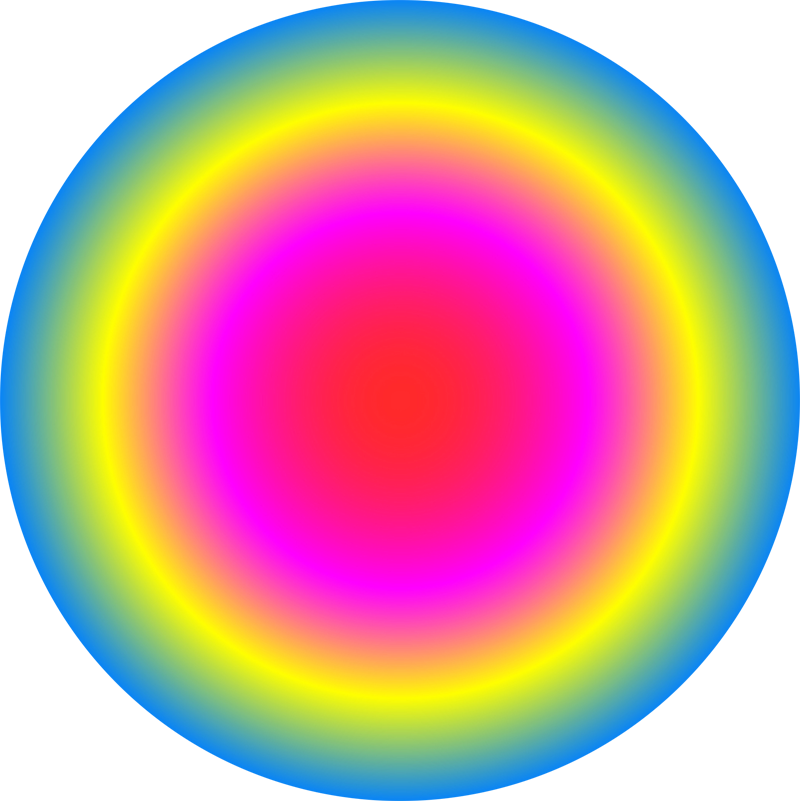# Conor Lawless

## Scientific Computing

data science, dynamic simulation modelling, genomics, interactive visualisation, dashboards, image & video analysis
e: cnr.lwlss@gmail.com
t: @cnrlwlss

## Exactly when do days begin and end?

If you live anywhere away from the equator, you’ll be familiar with the fact that days are short in winter and long in the summer. There’s even a slight effect at the equator, but the farther away from the equator (e.g. the farther north you live in Europe) the more extreme this effect is. At the poles, there is a period in the summertime when the sun never sets, and similarly in winter, there is a period when the sun never rises. ... Read more

## Translating maths into code: writing the tattoo function in Python

In a previous post I discussed a mathematical function which represents a range of different list-comprehensions and how their output can be visualised. Here I’ll describe in detail how we can evaluate this function on a computer, using the Python programming language as an example. Here is the mathematical notation for the tattoo function $t$ again: $$t(\theta, p) = \left\{ \sum_{k=-1}^{n}e^{\frac{2 \pi i k^p}{\theta}} \mid n \in -1,\dots,\theta \right\}$$ What should we expect from mathematical expressions in code? ... Read more

## Mathematical art: tattoos & list-comprehensions

In 2007, as part of a series of collaborations with the visual artist James Johnson-Perkins, I made a video demonstrating the diversity of output possible from a fairly simple mathematical function (see function $t$ below). The video was displayed on large, external, urban screens in Derby, Nottingham and Leicester as part of the ‘Do billboards dream of electric sheep?’ exhibition. The shapes displayed in each of the frames of the video were generated by passing the frame number to a function like $t$: ... Read more

## Interactive plots for comparing simulated output from simulation models: scientific computing & javascript

In a previous post , I used the interactive plot below to visualise & compare population growth curves simulated from three members of a family of models that share the same set of parameters. The user can adjust parameter values and explore the resulting changes in similarities and differences between simulations from the three models in real time. In this post I will discuss where dynamic visualisation of quantitative analysis is most useful and how the calculations underlying this analysis were carried out in JavaScript. ... Read more

## A family of mathematical models describing population growth

In this post, I will describe a family of three related mathematical models of population dynamics. Since their development, scientists have used these models to simulate the growth of a wide range of different types of populations including the growth of microbial cells, plant growth and the growth of human populations. Each of these three models are written down in the form of ordinary differential equations (ODEs) and are relatively straightforward to simulate from. ... Read more